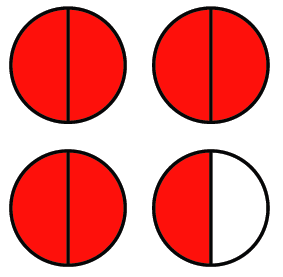### Home > ACC6 > Chapter 5 Unit 5 > Lesson CC1: 5.1.3 > Problem5-24

5-24.

Write each of the mixed numbers below as a fraction greater than one, and write each of the fractions greater than one as a mixed number. Include a diagram to explain each answer.

Refer to the Math Notes box at the bottom of the page for help.

1. $4 \frac { 1 } { 3 }$

This can be rewritten as:

$\frac{3}{3}+\frac{3}{3}+\frac{3}{3}+\frac{3}{3}+\frac{1}{3}$

1. $\frac{15}{4}$

This can be rewritten as:

$\frac{4}{4}+\frac{4}{4}+\frac{4}{4}+\frac{3}{4}$

1. $3 \frac { 1 } { 2 }$1. $\frac{15}{8}$

$1\frac{7}{8}$